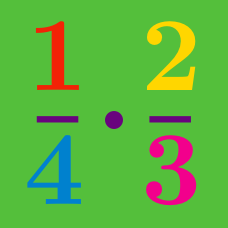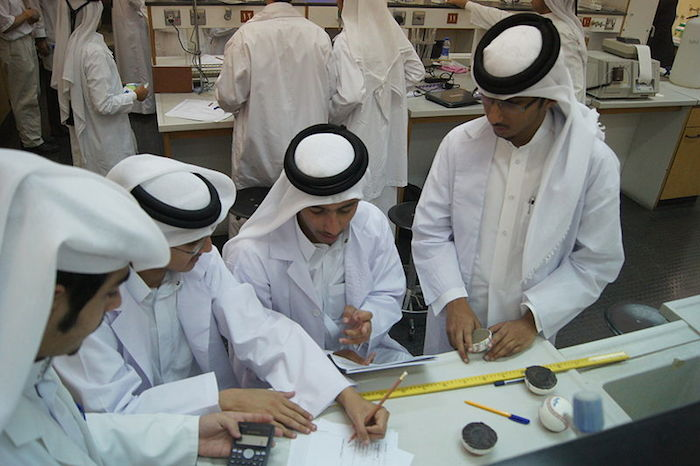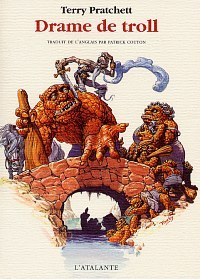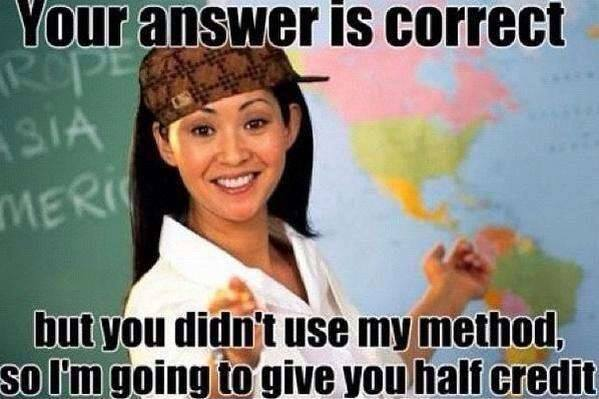Basic Mathematics

# Fractions: Level 1 Challenges

$\Large \frac {\color{#D61F06}1} {\color{#3D99F6}2} \times \frac {\color{#D61F06}2} {\color{#3D99F6}3} \times \frac {\color{#D61F06}3} {\color{#3D99F6}4} \times \frac {\color{#D61F06}4} {\color{#3D99F6}5} \times \ldots \times \frac { \color{#D61F06}9}{ \color{#3D99F6}{10}} = \ ?$Alex can solve a problem in 4 minutes. Brian can solve the same problem in $\frac{1}{5}$ of the time. How long does it take Brian to solve the problem?You are on your way to visit your grandma, and you have to cross seven bridges, each with a troll under them. You want to give your grandma two cakes. Whenever you cross a bridge, you must pay the troll half of your cakes. As he is nice, he will give you back one cake. How many cakes should you head out of the house with?

Simplify the expression below.

$\dfrac{999999\times999999}{1+2+3+4+5+6+7+8+9+8+7+6+5+4+3+2+1}$

TryTom was unhappy because he didn't get a full credit for this question:

Simplify $\frac{16}{64}$.

"Teacher, why I can't get full credit for this question?"

"Your answer is correct, but you didn't use the correct method, so I am going to give you half credit."

Actually what Tom did was simply cancel the two "6" that appear in the question as shown: $\frac{\overline{ab}}{\overline{bc}}=\frac{a}{c}$ $\frac{16}{64}=\frac{1\boxed{6}}{\boxed{6}4}=\frac{1}{4}$ Although Tom had gotten the correct answer, his method was wrong since 16 and 64 are 10+6 and 60+4 but not 1x6 and 6x4. Therefore, the "6" cannot be cancelled as shown in this method. But sometimes Tom's method is lucky and computes the correct solution.

For which of these fractions will Tom's method give a correct answer?

×